### 神经网络的工作原理### 前向传播

1. 为每个输入特征初始化系数θ。比方说，我们有100个训练例子。这意味着100行数据。在这种情况下，如果假设有10个输入特征，我们的输入矩阵的大小是100×10。现在确定$θ_1$的大小。行数需要与输入特征的数量相同。在这个例子中，是10。列数应该是你选择的隐藏层的大小。

1. 将输入特征X乘以相应的θ，然后添加一个偏置项。通过激活函数传递结果。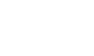g(z)是sigmoid激活函数：1. 为隐藏层初始化$\theta_2$。大小将是隐藏层的长度乘以输出类的数量。在这个例子中，下一层是输出层，因为我们没有更多的隐藏层。

1. 然后我们需要按照以前一样的流程。将θ和隐藏层相乘，通过sigmoid激活层得到预测输出。

### 反向传播

1. 首先，从原始输出y减去预测输出，这就是我们的$\delta_3$。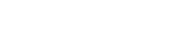1. 现在，计算$\theta_2$的梯度。将$\delta_3$乘以$\theta_2$。乘以“$a^2$”乘以“$1-a^2$”。在下面的公式中，“a”上的上标2表示第2层。请不要把它误解为平方。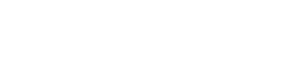1. 用训练样本数m计算没有正则化版本的梯度$\delta$。

### 训练网络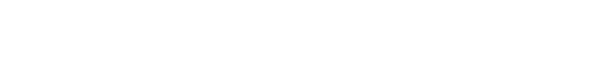### 神经网络的实现

https://github.com/rashida048…

1. 首先导入必要的包和数据集。

import pandas as pd
import numpy as np
xls = pd.ExcelFile('ex3d1.xlsx')
df = pd.read_excel(xls, 'X', header = None)y = pd.read_excel(xls, 'y', header=None)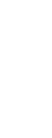1. 求输入输出变量的维数

df.shape
y.shape

1. 定义神经网络

hidden_layer = 25

y_arr = y.unique()#输出:
array([10,  1,  2,  3,  4,  5,  6,  7,  8,  9], dtype=int64)

1. 初始化θ和偏置

$\theta_1$的维度：第1层的大小x第2层的大小

$\theta_2$的维度：第2层的大小x第3层的大小

$\theta_1$的维度：400 x 25

$\theta_2$的维度：25×10

$b_1$的维度：第2层的大小(本例中为25)

$b_1$的维度：第3层的大小(本例中为10)

def randInitializeWeights(Lin, Lout):
epi = (6**1/2) / (Lin + Lout)**0.5
w = np.random.rand(Lout, Lin)*(2*epi) -epi
return w

hidden_layer = 25
output =10
theta1 = randInitializeWeights(len(df.T), hidden_layer)
theta2 = randInitializeWeights(hidden_layer, output)
theta = [theta1, theta2]

b1 = np.random.randn(25,)
b2 = np.random.randn(10,)

1. 实现前向传播def z_calc(X, theta):
return np.dot(X, theta.T)

def sigmoid(z):
return 1/(1+ np.exp(-z))

z1 =z_calc(df, theta1) + b1

a1 = sigmoid(z1)

a1是隐藏层。a1的形状是5000 x 25。重复相同的过程来计算第3层或输出层

z2 = z_calc(a1, theta2) + b2
a2 = sigmoid(z2)

a2的形状是5000 x 10。10列代表10个类。a2是我们的第3层或最终输出。如果在这个例子中有更多的隐藏层，在从一个层到另一个层的过程中会有更多的重复步骤。这种利用输入特征计算输出层的过程称为前向传播。

l = 3  #层数
b = [b1, b2]
def hypothesis(df, theta):
a = []
z = []
for i in range (0, l-1):
z1 = z_calc(df, theta[i]) + b[i]
out = sigmoid(z1)
a.append(out)
z.append(z1)
df = out
return out, a, z

1. 实现反向传播

y1 = np.zeros([len(df), len(y_arr)])
y1 = pd.DataFrame(y1)
for i in range(0, len(y_arr)):
for j in range(0, len(y1)):
if y[j] == y_arr[i]:
y1.iloc[j, i] = 1
else:
y1.iloc[j, i] = 0
y1.head()

del3 = y1-a2del2 = np.dot(del3, theta2) * a1*(1 - a1)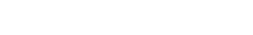def sigmoid_grad(z):
return sigmoid(z)*(1 - sigmoid(z))theta1 = np.dot(del2.T, pd.DataFrame(a1)) * 0.003
theta2 = np.dot(del3.T, pd.DataFrame(a2)) * 0.003

def cost_function(y, y_calc, l):
return (np.sum(np.sum(-np.log(y_calc)*y - np.log(1-y_calc)*(1-y))))/m

m = len(df)
def backpropagation(df, theta, y1, alpha):
out, a, z = hypothesis(df, theta)
delta = []
delta.append(y1-a[-1])
i = l - 2
while i > 0:
i -= 1
theta = np.dot(delta[-1].T, df) * alpha
for i in range(1, len(theta)):
theta[i] = np.dot(delta[-(i+1)].T, pd.DataFrame(a)) * alpha
out, a, z = hypothesis(df, theta)
cost = cost_function(y1, a[-1], 1)
return theta, cost

1. 训练网络

theta1 = randInitializeWeights(len(df.T), hidden_layer)
theta2 = randInitializeWeights(hidden_layer, output)
theta = [theta1, theta2]
cost_list = []
for i in range(20):
theta, cost= backpropagation(df, theta, y1, 0.003)
cost_list.append(cost)
cost_list

1. 预测输出并计算精度

out, a, z = hypothesis(df, theta)

accuracy= 0
for i in range(0, len(out)):
for j in range(0, len(out[i])):
if out[i][j] >= 0.5 and y1.iloc[i, j] == 1:
accuracy += 1
accuracy/len(df)

https://github.com/rashida048…